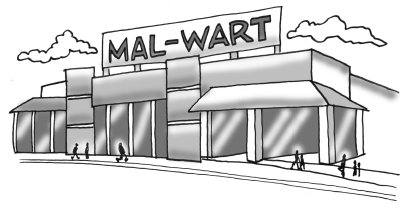### Home > CCA2 > Chapter B > Lesson B.2.3 > ProblemB-105

B-105.After noon, the number of people in Mal-Wart grows steadily until 6:00 p.m. If the equation $y = 228 + 58x$ represents the number of people in the store $x$ hours after noon:

1. How many people were in the store at noon?

Find the starting value in the equation.

1. At what rate is the number of shoppers growing?

Find the growth rate in the equation.

2. When were there $402$ shoppers in the store?

Set the equation equal to $402$ and solve for $x$.

Use the eTool below to solve the parts in the problem.
Click on the link at right for the full eTool version: B-105 HW eTool (Desmos)